Скачать презентацию Concep Test Power Points Chapter 2 Physics Principles

0cf12d27952aab6116f8129aeebc6a4a.ppt

• Количество слайдов: 51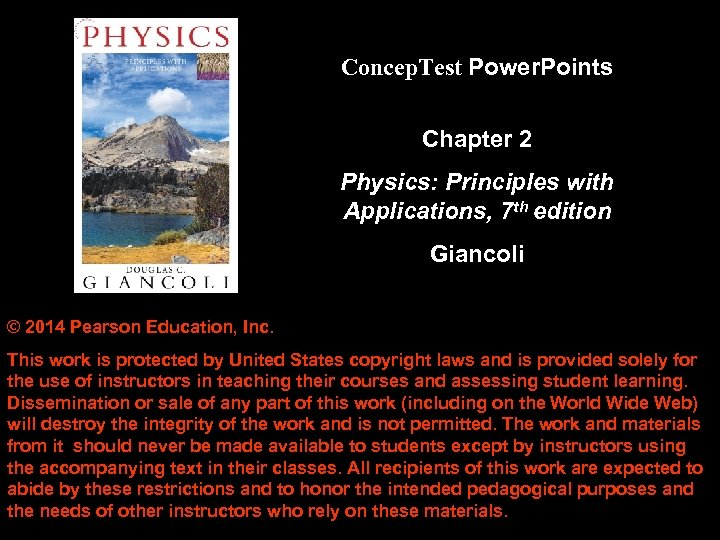Concep. Test Power. Points Chapter 2 Physics: Principles with Applications, 7 th edition Giancoli © 2014 Pearson Education, Inc. This work is protected by United States copyright laws and is provided solely for the use of instructors in teaching their courses and assessing student learning. Dissemination or sale of any part of this work (including on the World Wide Web) will destroy the integrity of the work and is not permitted. The work and materials from it should never be made available to students except by instructors using the accompanying text in their classes. All recipients of this work are expected to abide by these restrictions and to honor the intended pedagogical purposes and the needs of other instructors who rely on these materials.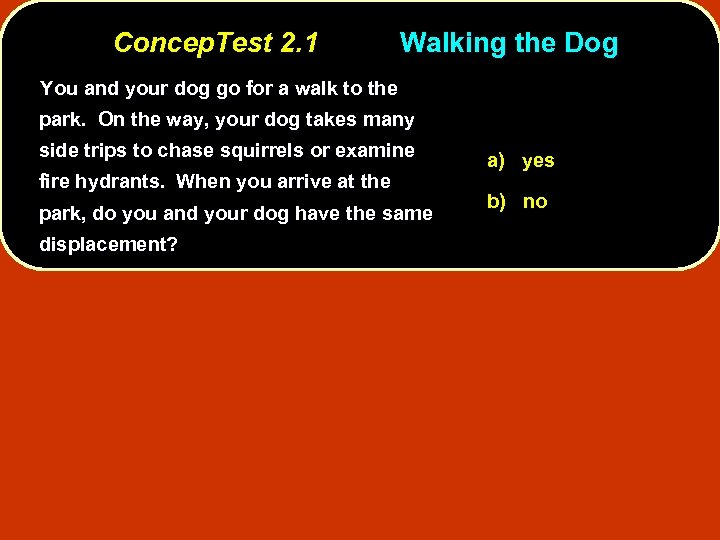Concep. Test 2. 1 Walking the Dog You and your dog go for a walk to the park. On the way, your dog takes many side trips to chase squirrels or examine fire hydrants. When you arrive at the park, do you and your dog have the same displacement? a) yes b) no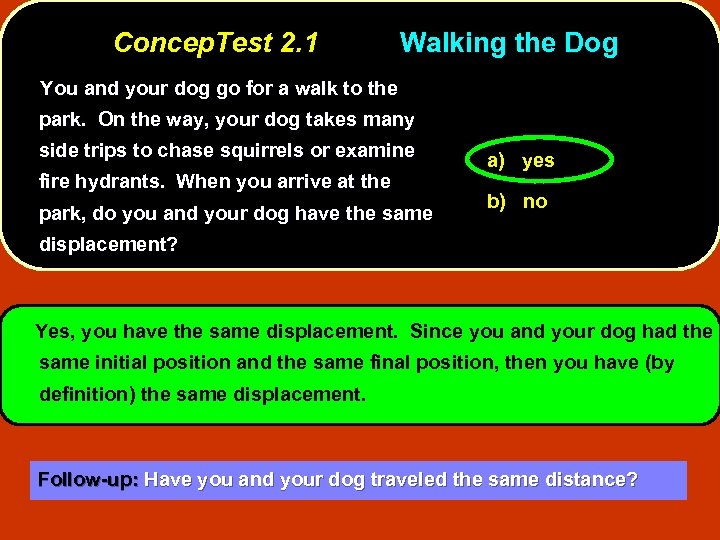Concep. Test 2. 1 Walking the Dog You and your dog go for a walk to the park. On the way, your dog takes many side trips to chase squirrels or examine fire hydrants. When you arrive at the park, do you and your dog have the same a) yes b) no displacement? Yes, you have the same displacement. Since you and your dog had the same initial position and the same final position, then you have (by definition) the same displacement. Follow-up: Have you and your dog traveled the same distance?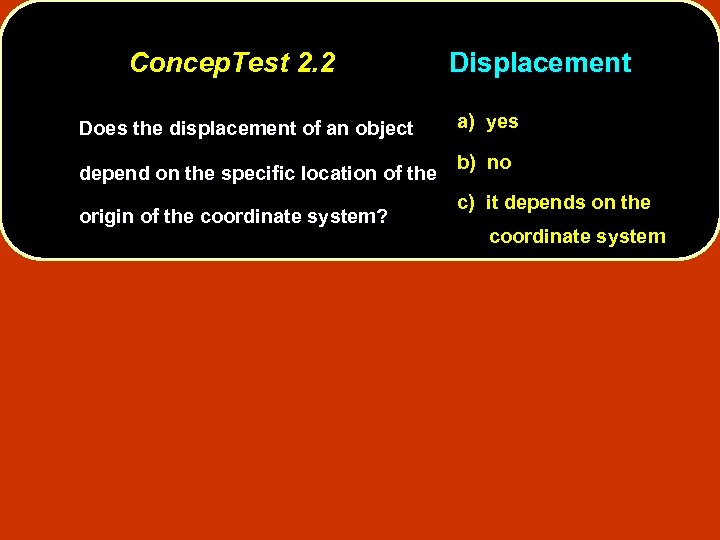Concep. Test 2. 2 Does the displacement of an object depend on the specific location of the origin of the coordinate system? Displacement a) yes b) no c) it depends on the coordinate system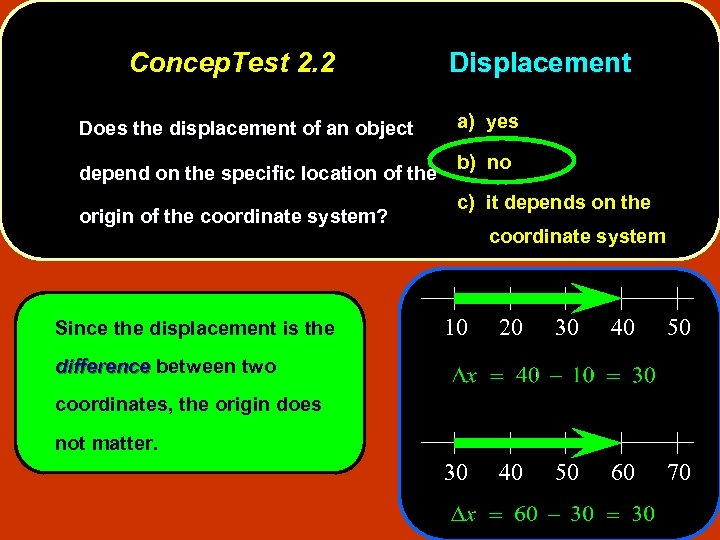Concep. Test 2. 2 Does the displacement of an object depend on the specific location of the origin of the coordinate system? Since the displacement is the Displacement a) yes b) no c) it depends on the coordinate system 10 20 30 40 50 60 70 difference between two coordinates, the origin does not matter.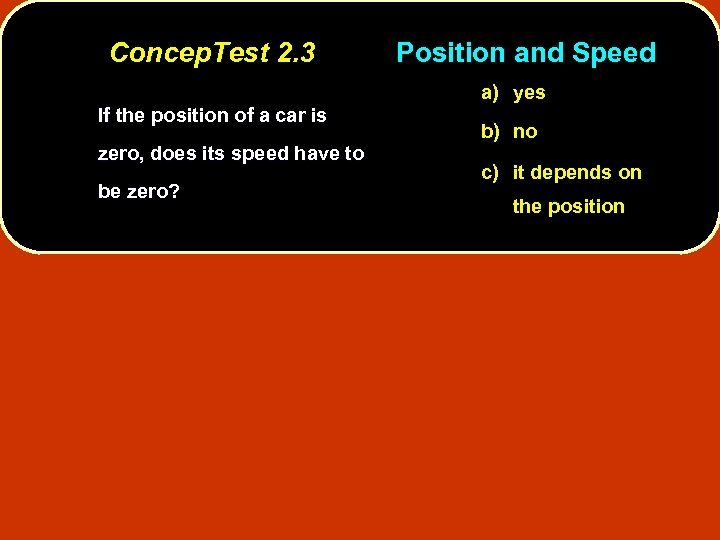Concep. Test 2. 3 Position and Speed a) yes If the position of a car is zero, does its speed have to be zero? b) no c) it depends on the position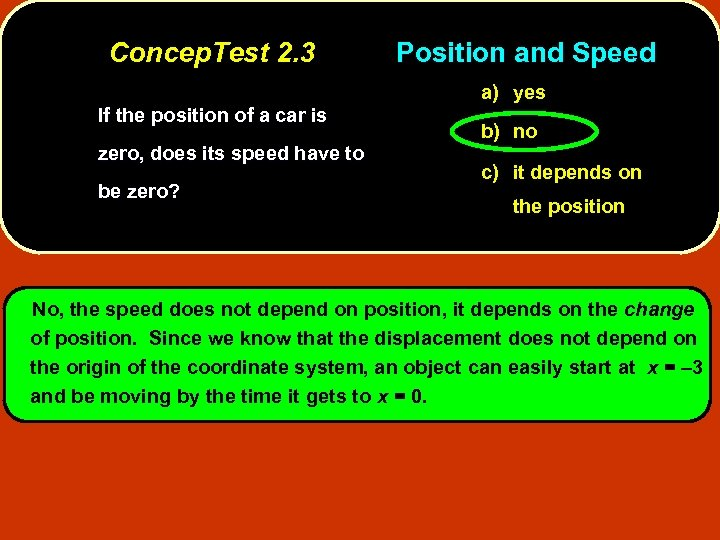Concep. Test 2. 3 Position and Speed a) yes If the position of a car is zero, does its speed have to be zero? b) no c) it depends on the position No, the speed does not depend on position, it depends on the change of position. Since we know that the displacement does not depend on the origin of the coordinate system, an object can easily start at x = – 3 and be moving by the time it gets to x = 0.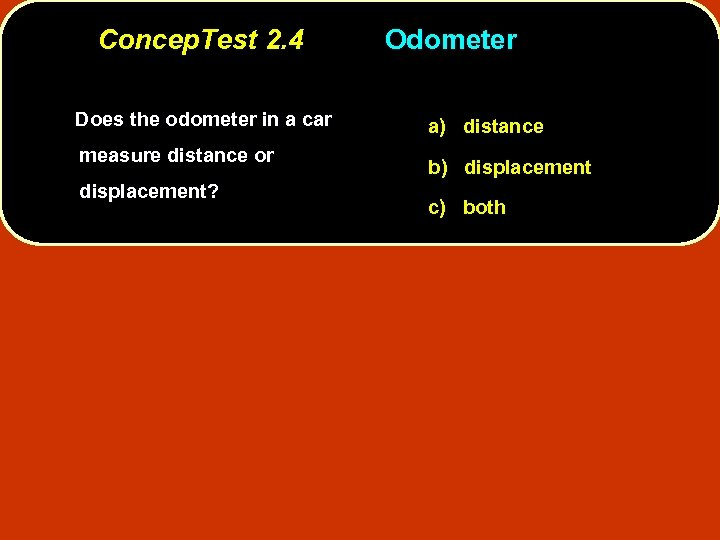Concep. Test 2. 4 Does the odometer in a car measure distance or displacement? Odometer a) distance b) displacement c) both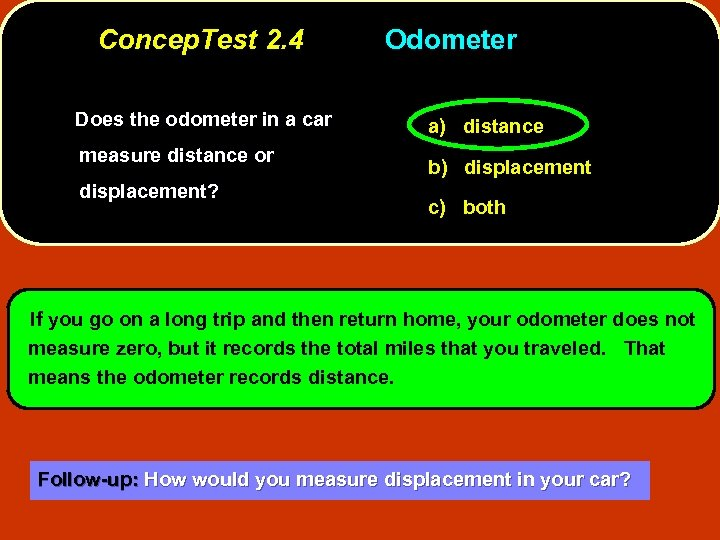Concep. Test 2. 4 Does the odometer in a car measure distance or displacement? Odometer a) distance b) displacement c) both If you go on a long trip and then return home, your odometer does not measure zero, but it records the total miles that you traveled. That means the odometer records distance. Follow-up: How would you measure displacement in your car?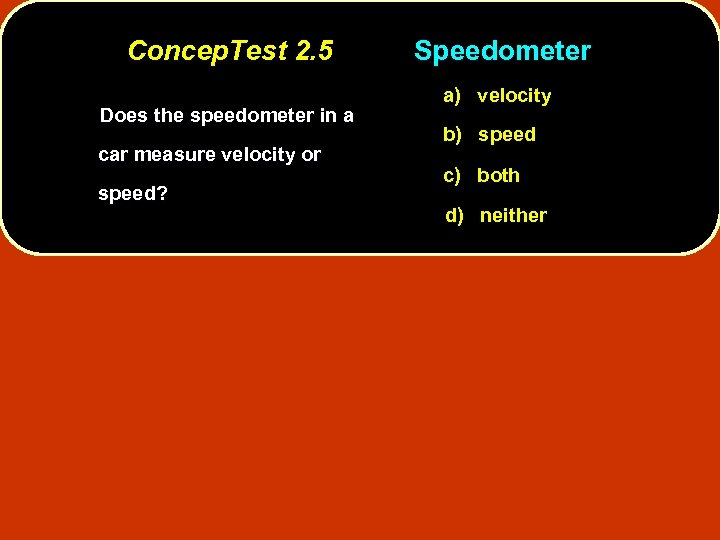Concep. Test 2. 5 Does the speedometer in a car measure velocity or speed? Speedometer a) velocity b) speed c) both d) neither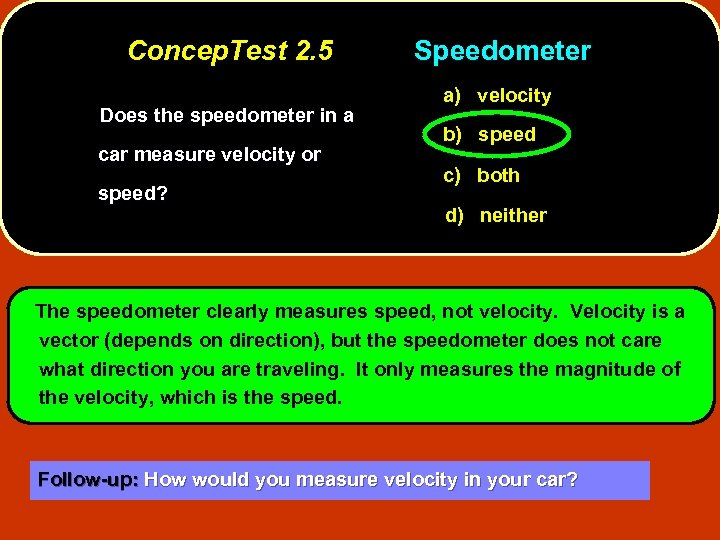Concep. Test 2. 5 Does the speedometer in a car measure velocity or speed? Speedometer a) velocity b) speed c) both d) neither The speedometer clearly measures speed, not velocity. Velocity is a vector (depends on direction), but the speedometer does not care what direction you are traveling. It only measures the magnitude of the velocity, which is the speed. Follow-up: How would you measure velocity in your car?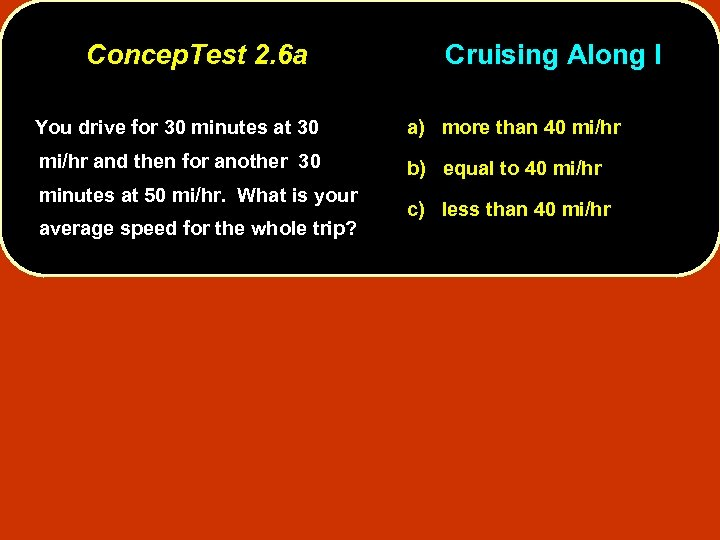Concep. Test 2. 6 a Cruising Along I You drive for 30 minutes at 30 a) more than 40 mi/hr and then for another 30 b) equal to 40 mi/hr minutes at 50 mi/hr. What is your average speed for the whole trip? c) less than 40 mi/hr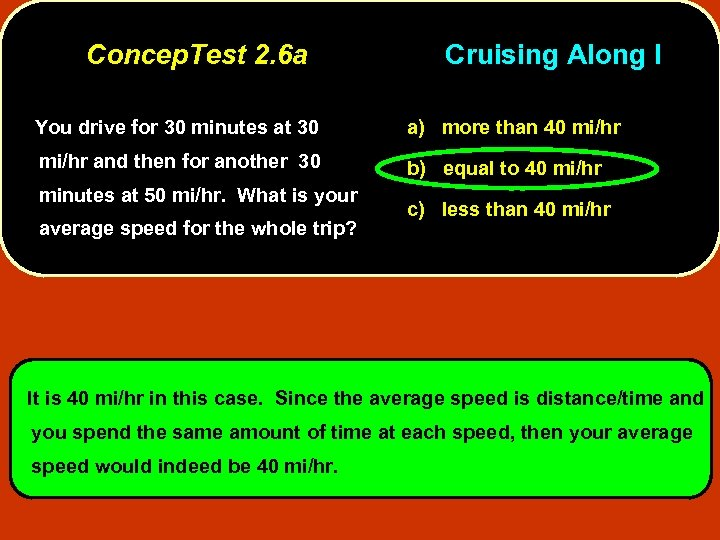Concep. Test 2. 6 a Cruising Along I You drive for 30 minutes at 30 a) more than 40 mi/hr and then for another 30 b) equal to 40 mi/hr minutes at 50 mi/hr. What is your average speed for the whole trip? c) less than 40 mi/hr It is 40 mi/hr in this case. Since the average speed is distance/time and you spend the same amount of time at each speed, then your average speed would indeed be 40 mi/hr.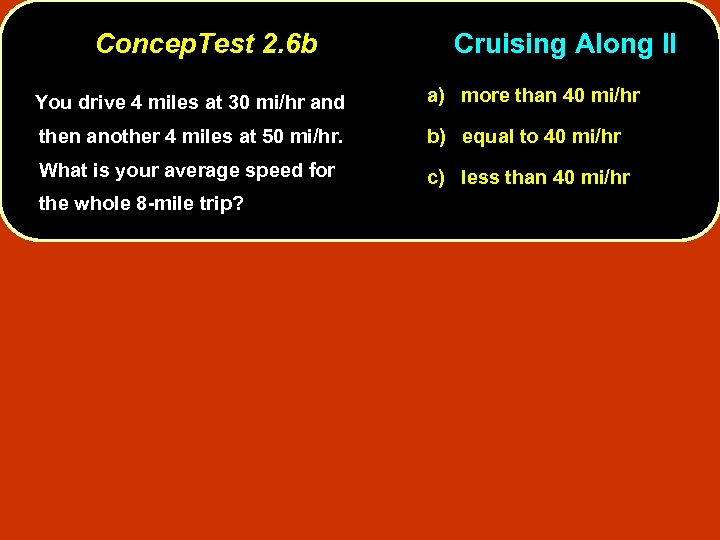Concep. Test 2. 6 b Cruising Along II You drive 4 miles at 30 mi/hr and a) more than 40 mi/hr then another 4 miles at 50 mi/hr. b) equal to 40 mi/hr What is your average speed for c) less than 40 mi/hr the whole 8 -mile trip?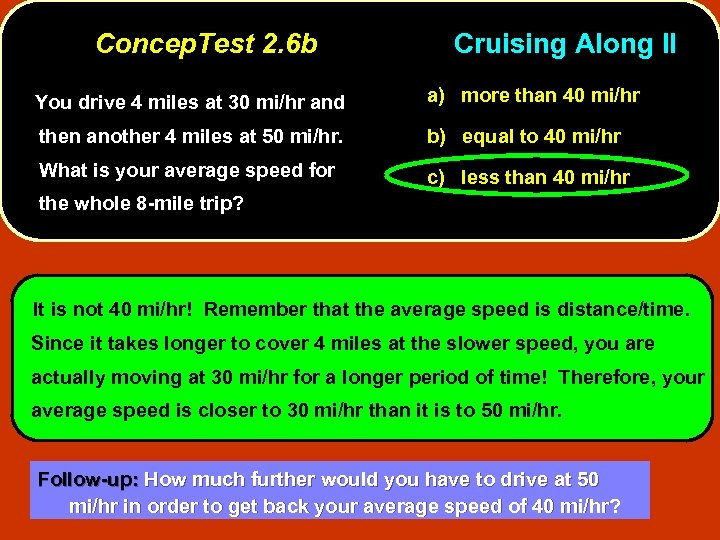Concep. Test 2. 6 b Cruising Along II You drive 4 miles at 30 mi/hr and a) more than 40 mi/hr then another 4 miles at 50 mi/hr. b) equal to 40 mi/hr What is your average speed for c) less than 40 mi/hr the whole 8 -mile trip? It is not 40 mi/hr! Remember that the average speed is distance/time. Since it takes longer to cover 4 miles at the slower speed, you are actually moving at 30 mi/hr for a longer period of time! Therefore, your average speed is closer to 30 mi/hr than it is to 50 mi/hr. Follow-up: How much further would you have to drive at 50 mi/hr in order to get back your average speed of 40 mi/hr?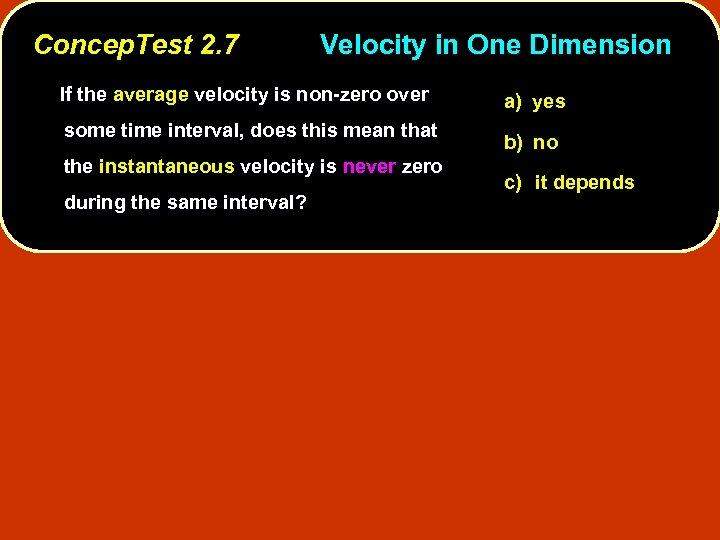Concep. Test 2. 7 Velocity in One Dimension If the average velocity is non-zero over some time interval, does this mean that the instantaneous velocity is never zero during the same interval? a) yes b) no c) it depends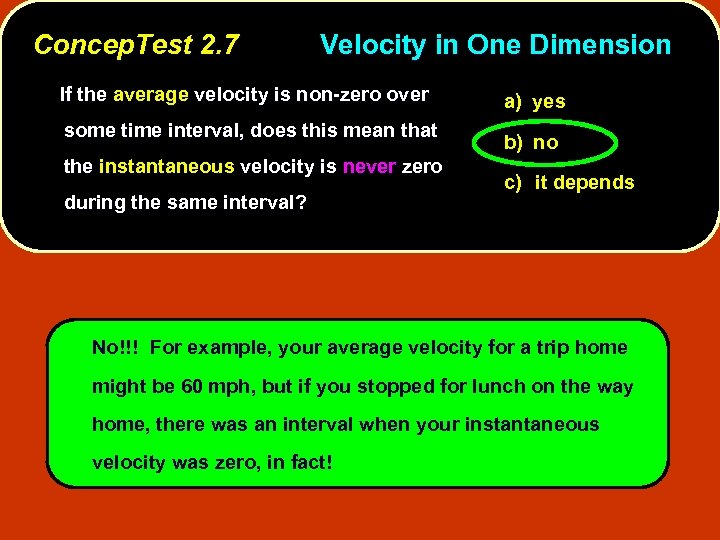Concep. Test 2. 7 Velocity in One Dimension If the average velocity is non-zero over some time interval, does this mean that the instantaneous velocity is never zero during the same interval? a) yes b) no c) it depends No!!! For example, your average velocity for a trip home might be 60 mph, but if you stopped for lunch on the way home, there was an interval when your instantaneous velocity was zero, in fact!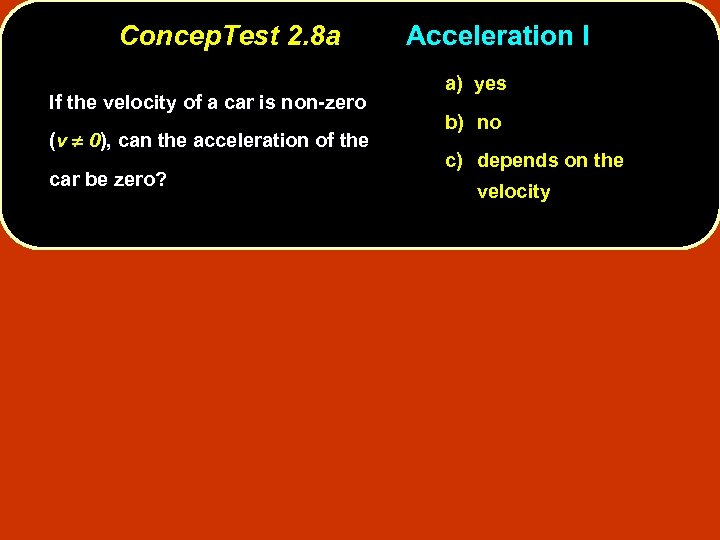Concep. Test 2. 8 a If the velocity of a car is non-zero (v ¹ 0), can the acceleration of the car be zero? Acceleration I a) yes b) no c) depends on the velocity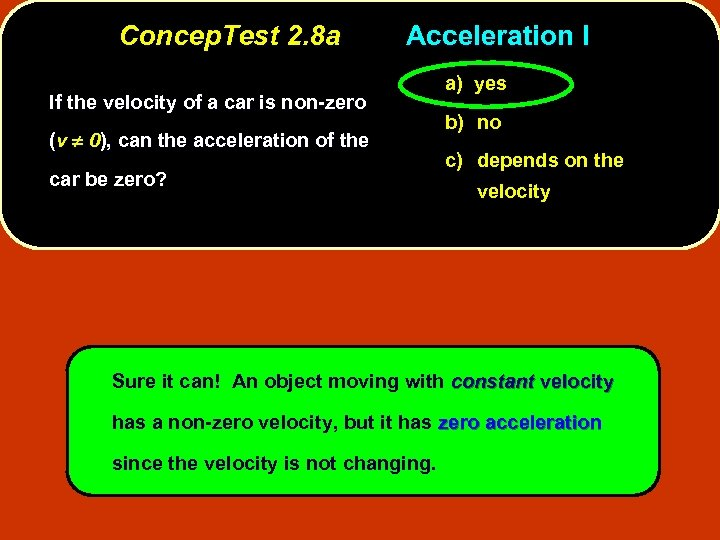Concep. Test 2. 8 a Acceleration I If the velocity of a car is non-zero (v ¹ 0), can the acceleration of the car be zero? a) yes b) no c) depends on the velocity Sure it can! An object moving with constant velocity has a non-zero velocity, but it has zero acceleration since the velocity is not changing.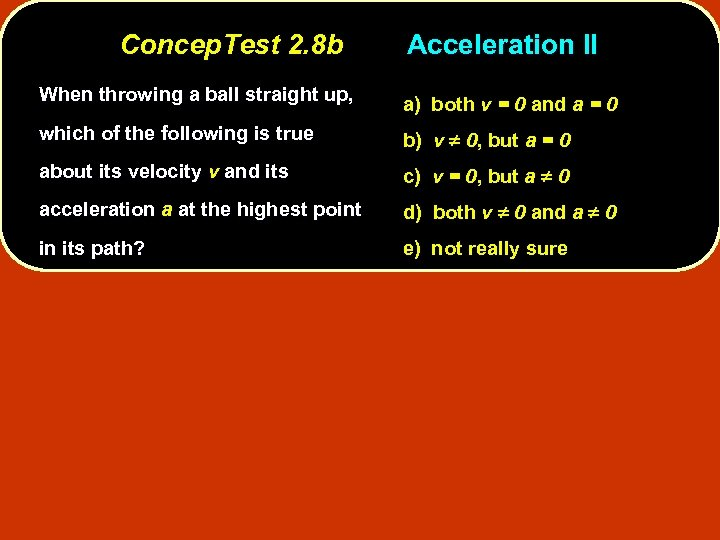Concep. Test 2. 8 b Acceleration II When throwing a ball straight up, a) both v = 0 and a = 0 which of the following is true b) v ¹ 0, but a = 0 about its velocity v and its c) v = 0, but a ¹ 0 acceleration a at the highest point d) both v ¹ 0 and a ¹ 0 in its path? e) not really sure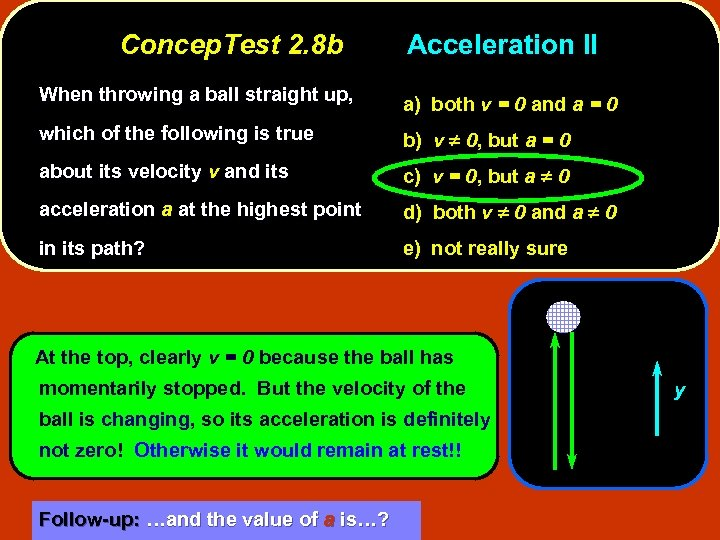Concep. Test 2. 8 b Acceleration II When throwing a ball straight up, a) both v = 0 and a = 0 which of the following is true b) v ¹ 0, but a = 0 about its velocity v and its c) v = 0, but a ¹ 0 acceleration a at the highest point d) both v ¹ 0 and a ¹ 0 in its path? e) not really sure At the top, clearly v = 0 because the ball has momentarily stopped. But the velocity of the ball is changing, so its acceleration is definitely not zero! Otherwise it would remain at rest!! Follow-up: …and the value of a is…? y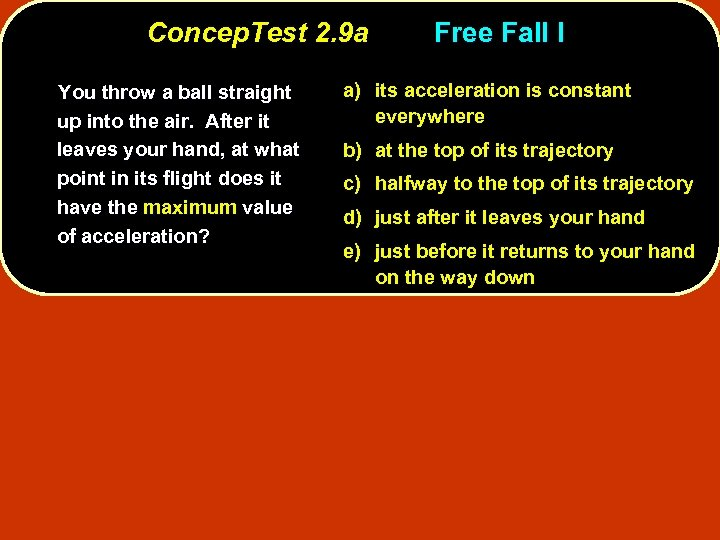Concep. Test 2. 9 a You throw a ball straight up into the air. After it leaves your hand, at what point in its flight does it have the maximum value of acceleration? Free Fall I a) its acceleration is constant everywhere b) at the top of its trajectory c) halfway to the top of its trajectory d) just after it leaves your hand e) just before it returns to your hand on the way down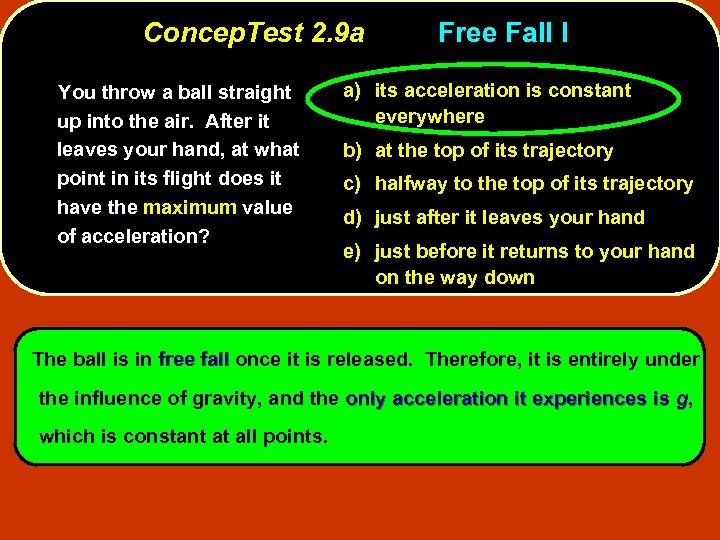Concep. Test 2. 9 a You throw a ball straight up into the air. After it leaves your hand, at what point in its flight does it have the maximum value of acceleration? Free Fall I a) its acceleration is constant everywhere b) at the top of its trajectory c) halfway to the top of its trajectory d) just after it leaves your hand e) just before it returns to your hand on the way down The ball is in free fall once it is released. Therefore, it is entirely under the influence of gravity, and the only acceleration it experiences is g, which is constant at all points.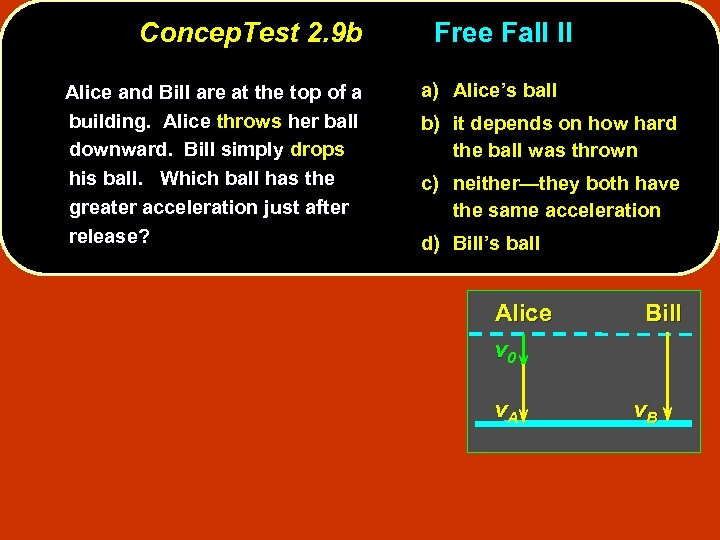Concep. Test 2. 9 b Alice and Bill are at the top of a building. Alice throws her ball downward. Bill simply drops his ball. Which ball has the greater acceleration just after release? Free Fall II a) Alice’s ball b) it depends on how hard the ball was thrown c) neither—they both have the same acceleration d) Bill’s ball Alice v 0 v. A Bill v. B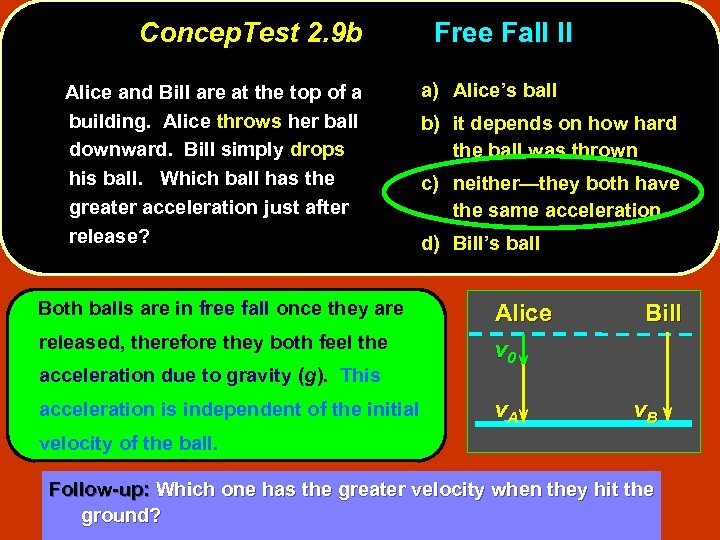Concep. Test 2. 9 b Alice and Bill are at the top of a building. Alice throws her ball downward. Bill simply drops his ball. Which ball has the greater acceleration just after release? Both balls are in free fall once they are released, therefore they both feel the acceleration due to gravity (g). This acceleration is independent of the initial Free Fall II a) Alice’s ball b) it depends on how hard the ball was thrown c) neither—they both have the same acceleration d) Bill’s ball Alice v 0 v. A Bill v. B velocity of the ball. Follow-up: Which one has the greater velocity when they hit the ground?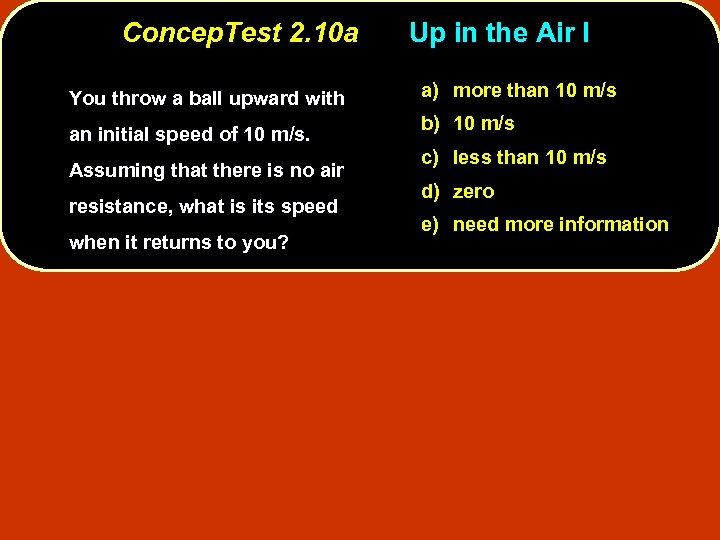Concep. Test 2. 10 a You throw a ball upward with an initial speed of 10 m/s. Assuming that there is no air resistance, what is its speed when it returns to you? Up in the Air I a) more than 10 m/s b) 10 m/s c) less than 10 m/s d) zero e) need more information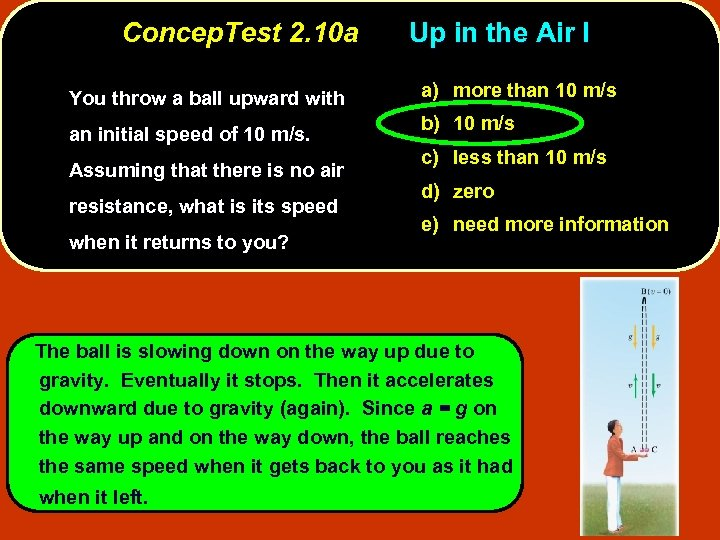Concep. Test 2. 10 a You throw a ball upward with an initial speed of 10 m/s. Assuming that there is no air resistance, what is its speed when it returns to you? Up in the Air I a) more than 10 m/s b) 10 m/s c) less than 10 m/s d) zero e) need more information The ball is slowing down on the way up due to gravity. Eventually it stops. Then it accelerates downward due to gravity (again). Since a = g on the way up and on the way down, the ball reaches the same speed when it gets back to you as it had when it left.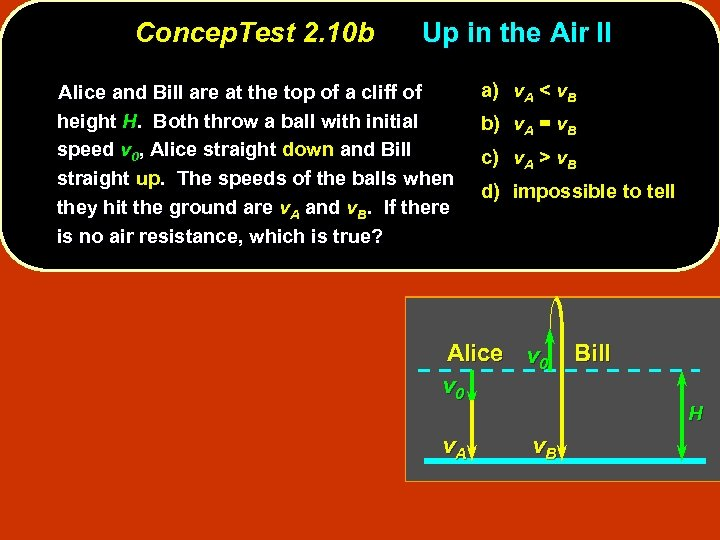Concep. Test 2. 10 b Up in the Air II Alice and Bill are at the top of a cliff of height H. Both throw a ball with initial speed v 0, Alice straight down and Bill straight up. The speeds of the balls when they hit the ground are v. A and v. B. If there is no air resistance, which is true? a) v. A < v. B b) v. A = v. B c) v. A > v. B d) impossible to tell Alice v 0 v. A v. B Bill H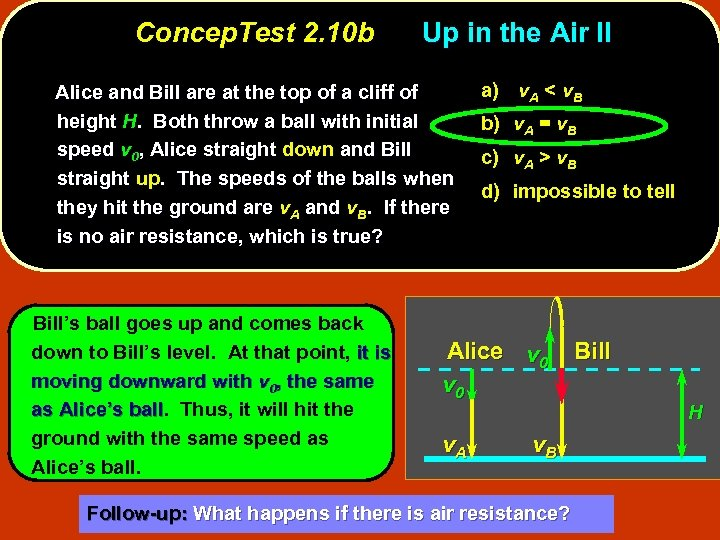Concep. Test 2. 10 b Up in the Air II Alice and Bill are at the top of a cliff of height H. Both throw a ball with initial speed v 0, Alice straight down and Bill straight up. The speeds of the balls when they hit the ground are v. A and v. B. If there is no air resistance, which is true? Bill’s ball goes up and comes back down to Bill’s level. At that point, it is moving downward with v 0, the same as Alice’s ball. Thus, it will hit the ball ground with the same speed as Alice’s ball. a) v. A < v. B b) v. A = v. B c) v. A > v. B d) impossible to tell Alice v 0 v. A v. B Follow-up: What happens if there is air resistance? Bill H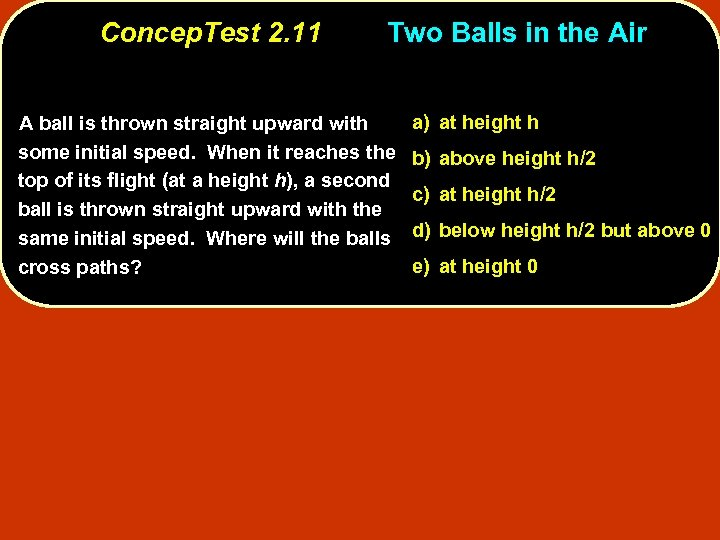Concep. Test 2. 11 Two Balls in the Air A ball is thrown straight upward with some initial speed. When it reaches the top of its flight (at a height h), a second ball is thrown straight upward with the same initial speed. Where will the balls cross paths? a) at height h b) above height h/2 c) at height h/2 d) below height h/2 but above 0 e) at height 0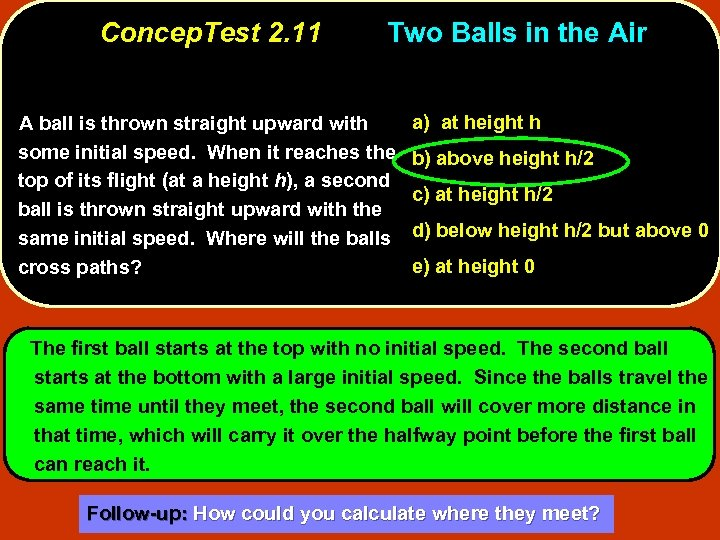Concep. Test 2. 11 Two Balls in the Air A ball is thrown straight upward with some initial speed. When it reaches the top of its flight (at a height h), a second ball is thrown straight upward with the same initial speed. Where will the balls cross paths? a) at height h b) above height h/2 c) at height h/2 d) below height h/2 but above 0 e) at height 0 The first ball starts at the top with no initial speed. The second ball starts at the bottom with a large initial speed. Since the balls travel the same time until they meet, the second ball will cover more distance in that time, which will carry it over the halfway point before the first ball can reach it. Follow-up: How could you calculate where they meet?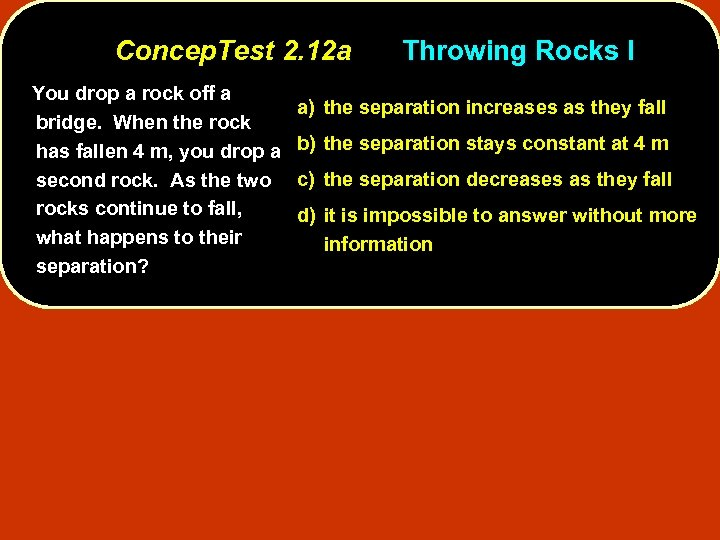Concep. Test 2. 12 a You drop a rock off a bridge. When the rock has fallen 4 m, you drop a second rock. As the two rocks continue to fall, what happens to their separation? Throwing Rocks I a) the separation increases as they fall b) the separation stays constant at 4 m c) the separation decreases as they fall d) it is impossible to answer without more information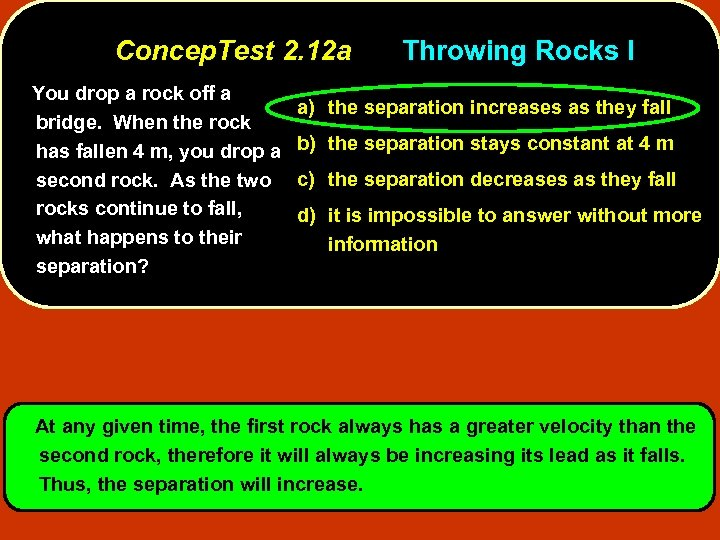Concep. Test 2. 12 a You drop a rock off a bridge. When the rock has fallen 4 m, you drop a second rock. As the two rocks continue to fall, what happens to their separation? Throwing Rocks I a) the separation increases as they fall b) the separation stays constant at 4 m c) the separation decreases as they fall d) it is impossible to answer without more information At any given time, the first rock always has a greater velocity than the second rock, therefore it will always be increasing its lead as it falls. Thus, the separation will increase.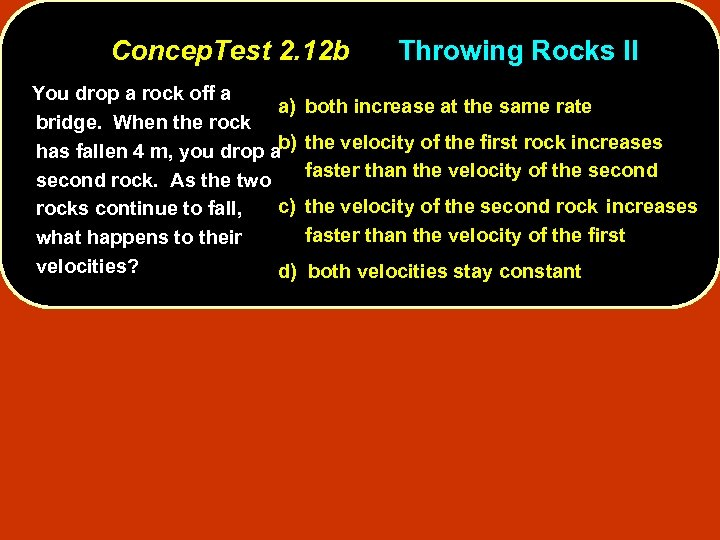Concep. Test 2. 12 b You drop a rock off a a) bridge. When the rock has fallen 4 m, you drop ab) second rock. As the two c) rocks continue to fall, what happens to their velocities? Throwing Rocks II both increase at the same rate the velocity of the first rock increases faster than the velocity of the second rock increases faster than the velocity of the first d) both velocities stay constant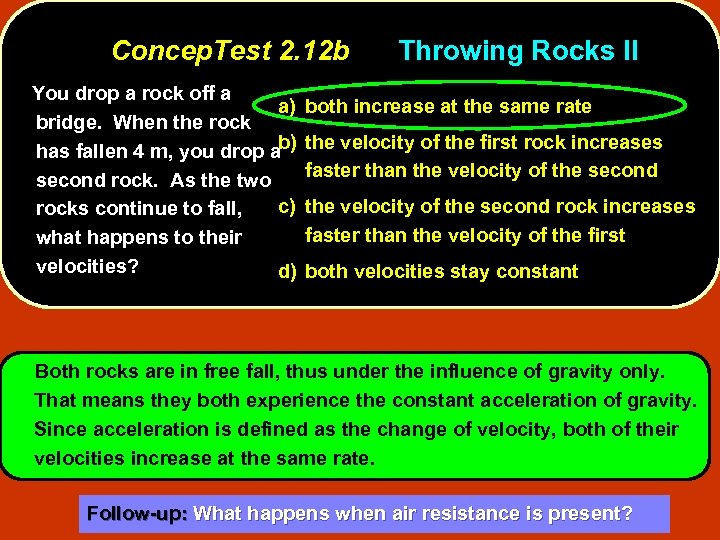Concep. Test 2. 12 b You drop a rock off a a) bridge. When the rock has fallen 4 m, you drop ab) second rock. As the two c) rocks continue to fall, what happens to their velocities? Throwing Rocks II both increase at the same rate the velocity of the first rock increases faster than the velocity of the second rock increases faster than the velocity of the first d) both velocities stay constant Both rocks are in free fall, thus under the influence of gravity only. That means they both experience the constant acceleration of gravity. Since acceleration is defined as the change of velocity, both of their velocities increase at the same rate. Follow-up: What happens when air resistance is present?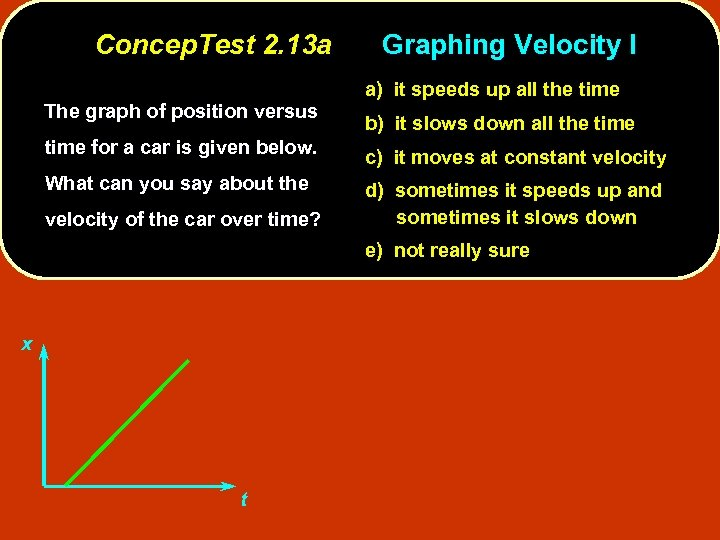Concep. Test 2. 13 a The graph of position versus Graphing Velocity I a) it speeds up all the time b) it slows down all the time for a car is given below. c) it moves at constant velocity What can you say about the d) sometimes it speeds up and sometimes it slows down velocity of the car over time? e) not really sure x t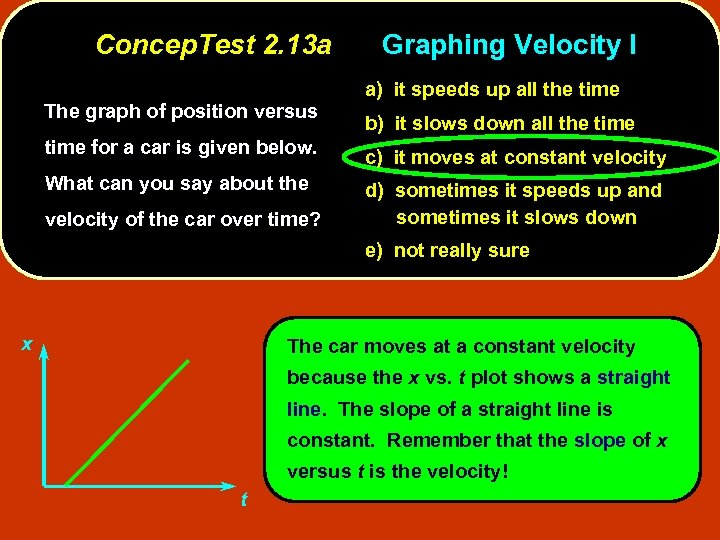Concep. Test 2. 13 a The graph of position versus Graphing Velocity I a) it speeds up all the time b) it slows down all the time for a car is given below. c) it moves at constant velocity What can you say about the d) sometimes it speeds up and sometimes it slows down velocity of the car over time? e) not really sure x The car moves at a constant velocity because the x vs. t plot shows a straight line. The slope of a straight line is constant. Remember that the slope of x versus t is the velocity! t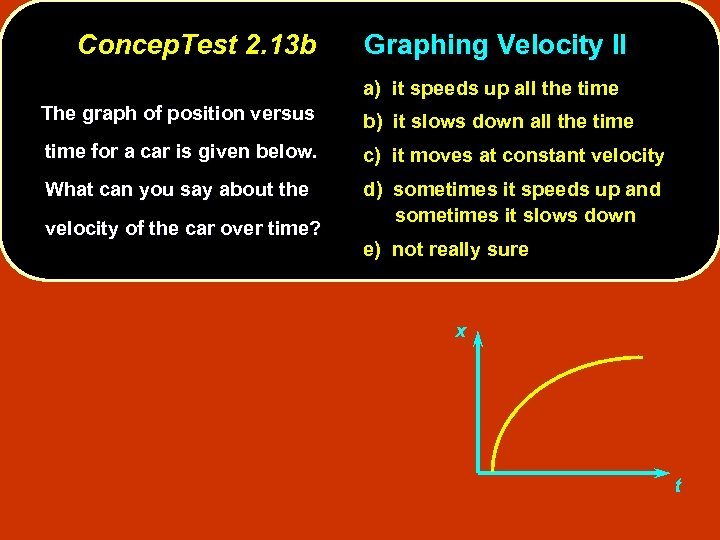Concep. Test 2. 13 b Graphing Velocity II a) it speeds up all the time The graph of position versus b) it slows down all the time for a car is given below. c) it moves at constant velocity What can you say about the d) sometimes it speeds up and sometimes it slows down velocity of the car over time? e) not really sure x t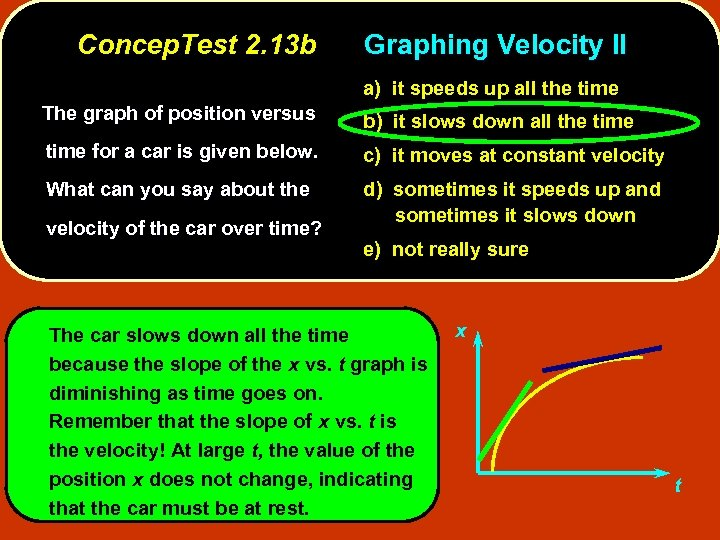Concep. Test 2. 13 b Graphing Velocity II a) it speeds up all the time The graph of position versus b) it slows down all the time for a car is given below. c) it moves at constant velocity What can you say about the d) sometimes it speeds up and sometimes it slows down velocity of the car over time? e) not really sure The car slows down all the time because the slope of the x vs. t graph is diminishing as time goes on. Remember that the slope of x vs. t is the velocity! At large t, the value of the position x does not change, indicating that the car must be at rest. x t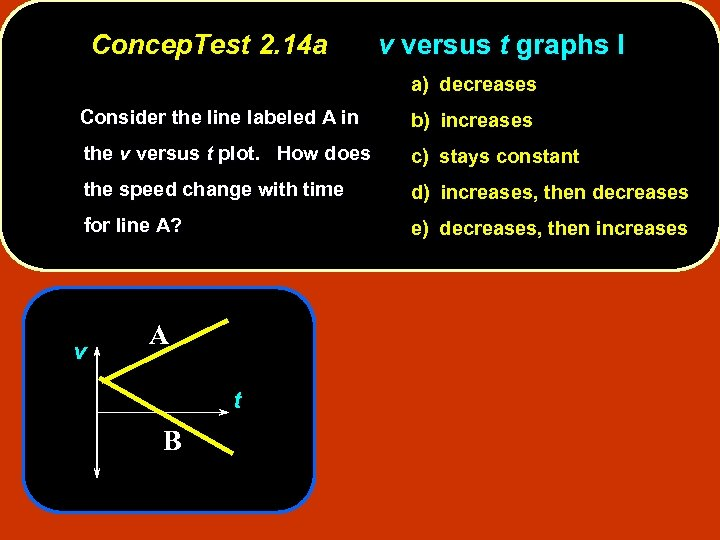Concep. Test 2. 14 a v versus t graphs I a) decreases Consider the line labeled A in b) increases the v versus t plot. How does c) stays constant the speed change with time d) increases, then decreases for line A? e) decreases, then increases v A t B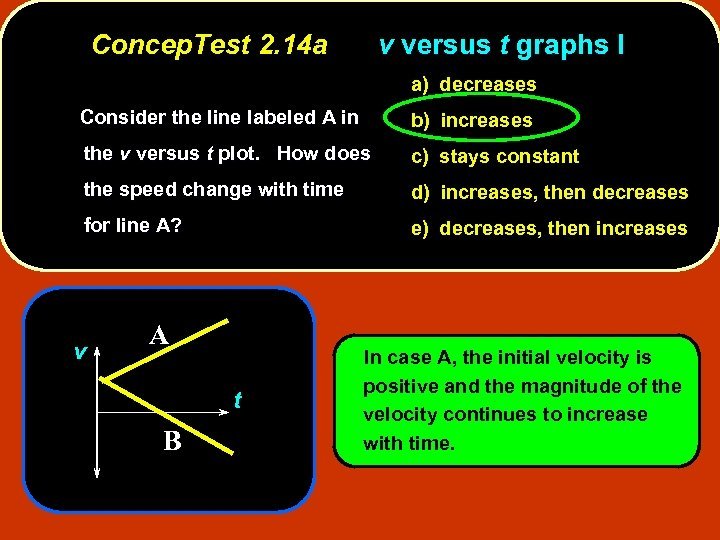Concep. Test 2. 14 a v versus t graphs I a) decreases Consider the line labeled A in b) increases the v versus t plot. How does c) stays constant the speed change with time d) increases, then decreases for line A? e) decreases, then increases v A t B In case A, the initial velocity is positive and the magnitude of the velocity continues to increase with time.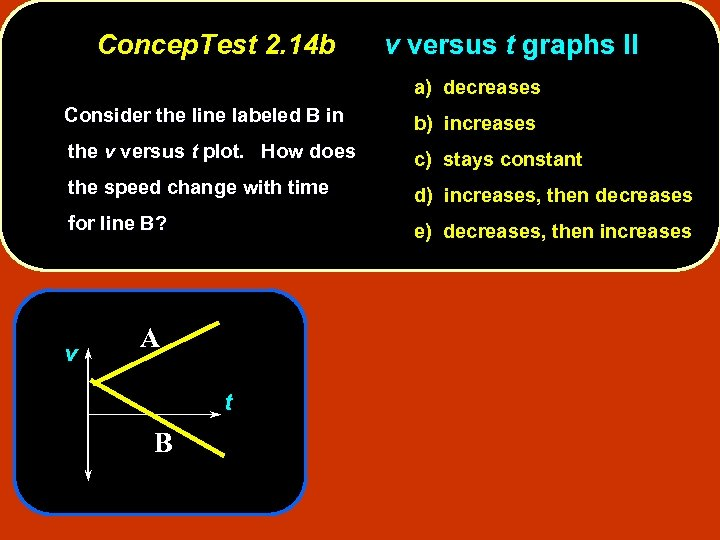Concep. Test 2. 14 b v versus t graphs II a) decreases Consider the line labeled B in b) increases the v versus t plot. How does c) stays constant the speed change with time d) increases, then decreases for line B? e) decreases, then increases v A t B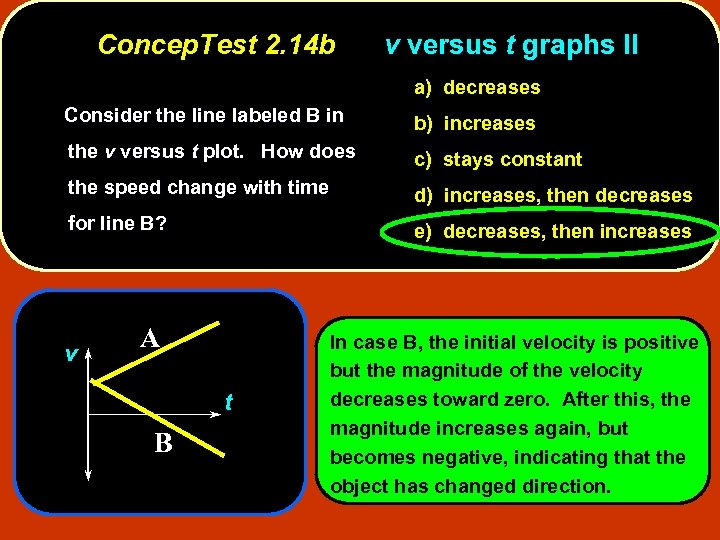Concep. Test 2. 14 b v versus t graphs II a) decreases Consider the line labeled B in b) increases the v versus t plot. How does c) stays constant the speed change with time d) increases, then decreases for line B? e) decreases, then increases v A t B In case B, the initial velocity is positive but the magnitude of the velocity decreases toward zero. After this, the magnitude increases again, but becomes negative, indicating that the object has changed direction.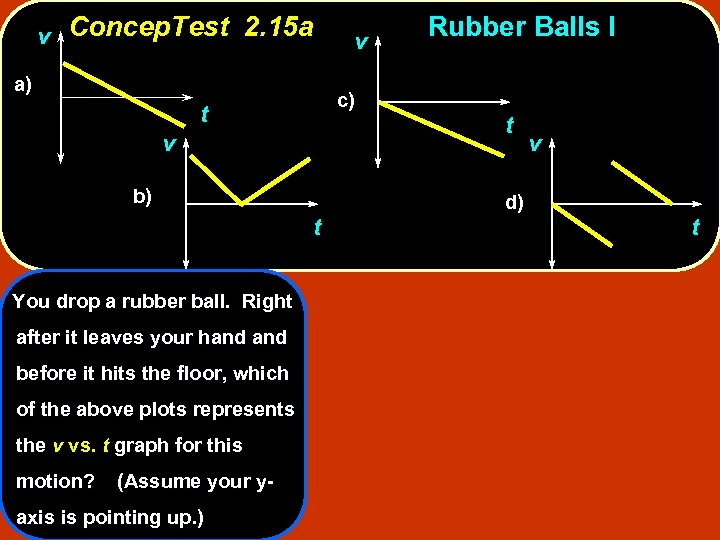v Concep. Test 2. 15 a a) c) t v b) t You drop a rubber ball. Right after it leaves your hand before it hits the floor, which of the above plots represents the v vs. t graph for this motion? (Assume your y- axis is pointing up. ) v Rubber Balls I t d) v t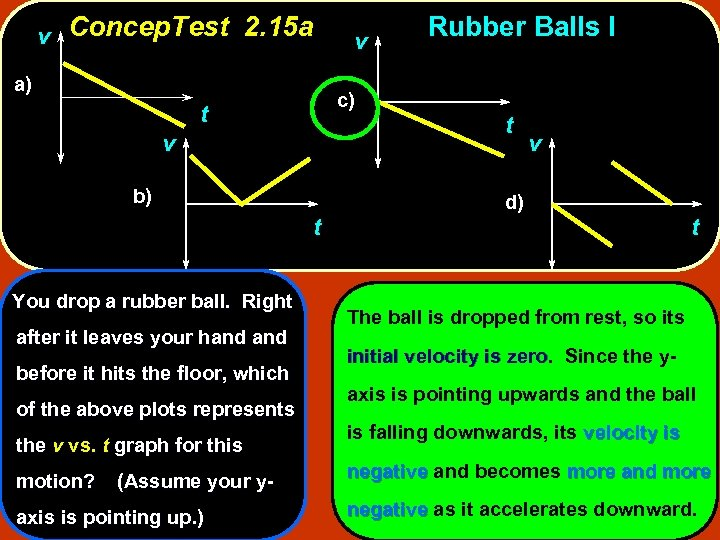v Concep. Test 2. 15 a a) c) t v b) t You drop a rubber ball. Right after it leaves your hand before it hits the floor, which of the above plots represents the v vs. t graph for this motion? (Assume your y- axis is pointing up. ) v Rubber Balls I t v d) t The ball is dropped from rest, so its initial velocity is zero. Since the yzero axis is pointing upwards and the ball is falling downwards, its velocity is negative and becomes more and more negative as it accelerates downward.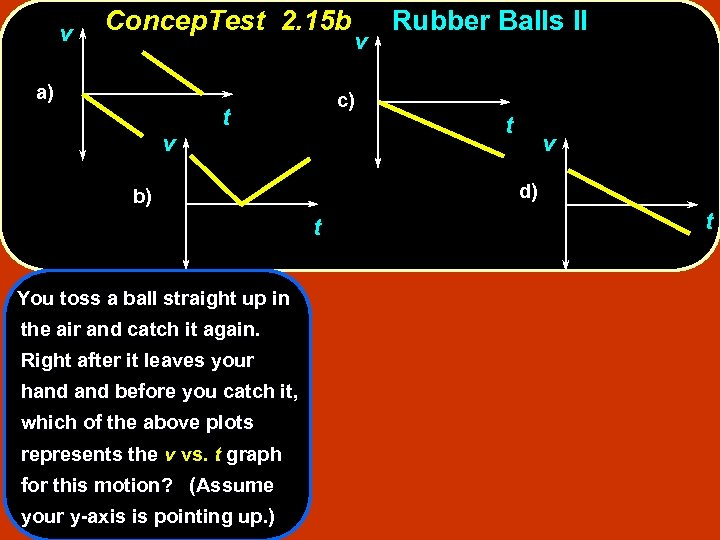v Concep. Test 2. 15 b a) v c) t t the air and catch it again. Right after it leaves your hand before you catch it, which of the above plots represents the v vs. t graph for this motion? (Assume your y-axis is pointing up. ) t v d) b) You toss a ball straight up in v Rubber Balls II t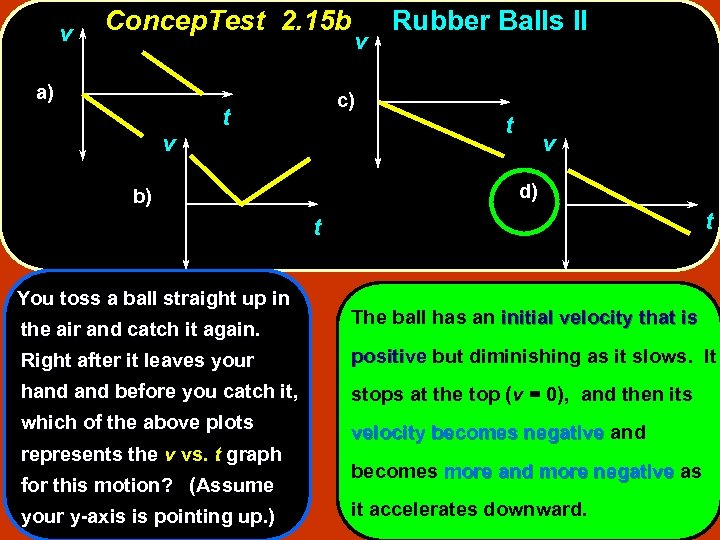v Concep. Test 2. 15 b a) v v c) t Rubber Balls II t v d) b) t t You toss a ball straight up in the air and catch it again. The ball has an initial velocity that is Right after it leaves your positive but diminishing as it slows. It hand before you catch it, stops at the top (v = 0), and then its which of the above plots velocity becomes negative and represents the v vs. t graph for this motion? (Assume your y-axis is pointing up. ) becomes more and more negative as it accelerates downward.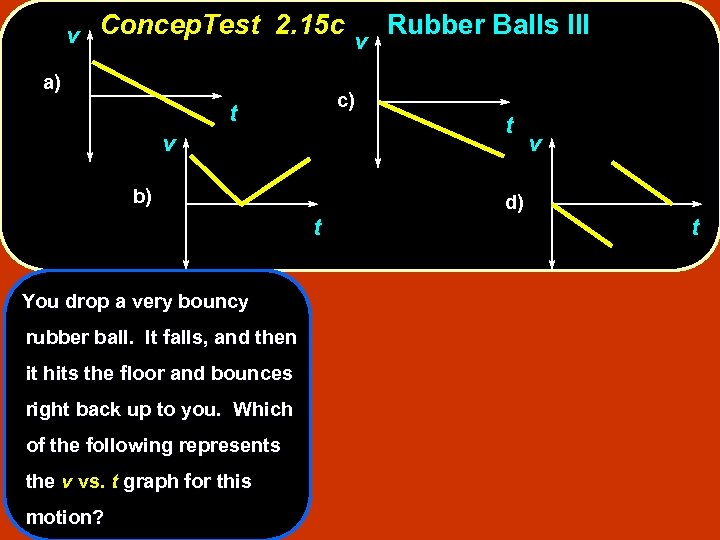v Concep. Test 2. 15 c v Rubber Balls III a) c) t v b) t You drop a very bouncy rubber ball. It falls, and then it hits the floor and bounces right back up to you. Which of the following represents the v vs. t graph for this motion? t d) v t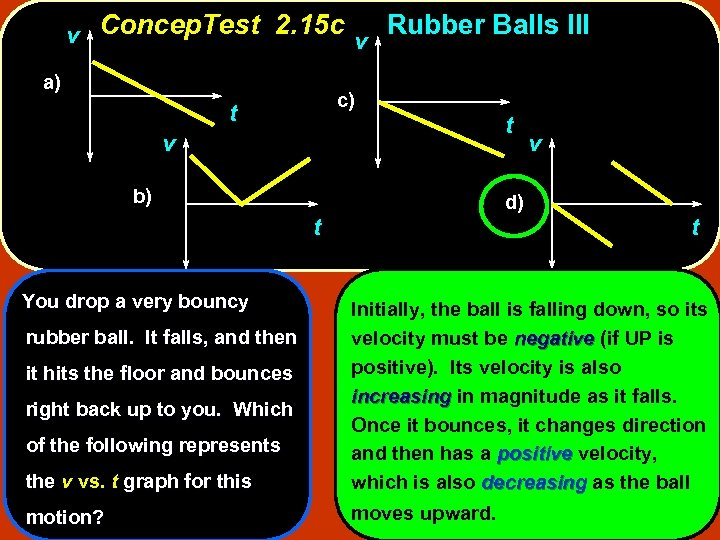v Concep. Test 2. 15 c v Rubber Balls III a) c) t v b) d) t You drop a very bouncy t v t the v vs. t graph for this Initially, the ball is falling down, so its velocity must be negative (if UP is positive). Its velocity is also increasing in magnitude as it falls. Once it bounces, it changes direction and then has a positive velocity, which is also decreasing as the ball motion? moves upward. rubber ball. It falls, and then it hits the floor and bounces right back up to you. Which of the following represents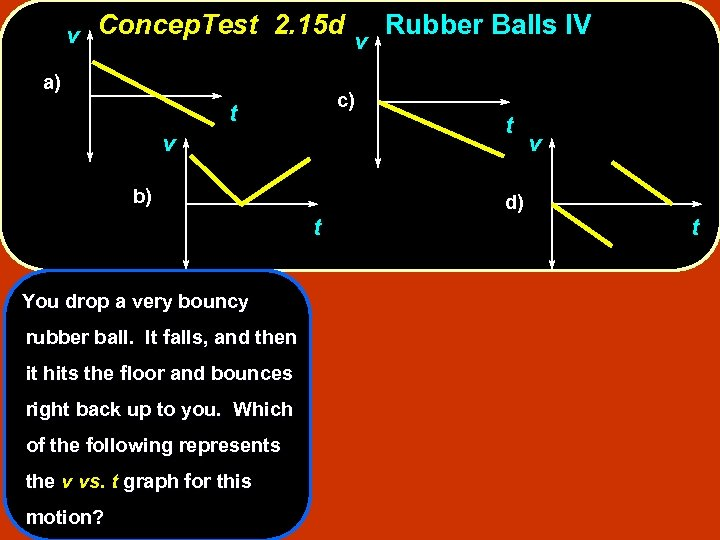v Concep. Test 2. 15 d v Rubber Balls IV a) c) t v b) t You drop a very bouncy rubber ball. It falls, and then it hits the floor and bounces right back up to you. Which of the following represents the v vs. t graph for this motion? t d) v t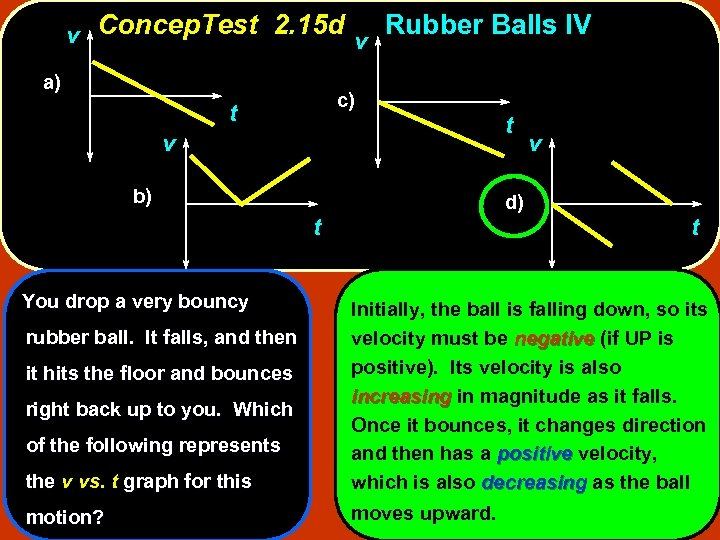v Concep. Test 2. 15 d v Rubber Balls IV a) c) t v b) d) t You drop a very bouncy t v t the v vs. t graph for this Initially, the ball is falling down, so its velocity must be negative (if UP is positive). Its velocity is also increasing in magnitude as it falls. Once it bounces, it changes direction and then has a positive velocity, which is also decreasing as the ball motion? moves upward. rubber ball. It falls, and then it hits the floor and bounces right back up to you. Which of the following represents